## 4.3.2 Explicitly Modeling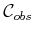: The Translational Case

It is important to understand how to construct a representation of. In some algorithms, especially the combinatorial methods of Chapter 6, this represents an important first step to solving the problem. In other algorithms, especially the sampling-based planning algorithms of Chapter 5, it helps to understand why such constructions are avoided due to their complexity.

The simplest case for characterizingis when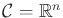for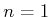,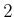, and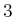, and the robot is a rigid body that is restricted to translation only. Under these conditions,can be expressed as a type of convolution. For any two sets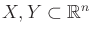, let their Minkowski difference4.10 be defined as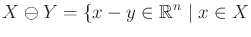and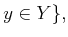(4.37)

in which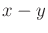is just vector subtraction on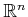. The Minkowski difference betweenand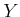can also be considered as the Minkowski sum ofand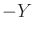. The Minkowski sum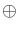is obtained by simply adding elements ofandin (4.37), as opposed to subtracting them. The setis obtained by replacing each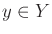by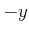.

In terms of the Minkowski difference,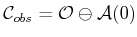. To see this, it is helpful to consider a one-dimensional example.

Example 4..13 (One-Dimensional C-Space Obstacle)   In Figure 4.12, both the robot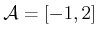and obstacle region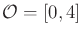are intervals in a one-dimensional world,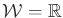. The negation,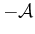, of the robot is shown as the interval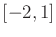. Finally, by applying the Minkowski sum to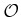and, the C-space obstacle,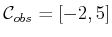, is obtained.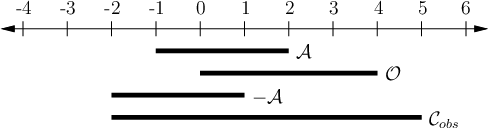The Minkowski difference is often considered as a convolution. It can even be defined to appear the same as studied in differential equations and system theory. For a one-dimensional example, let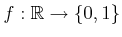be a function such that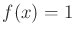if and only if. Similarly, let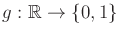be a function such that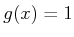if and only if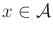. The convolution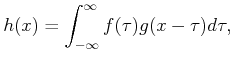(4.38)

yields a function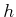, for which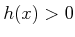if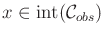, and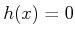otherwise.

Subsections
Steven M LaValle 2020-08-14